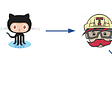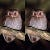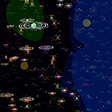# Custom Bezier Curve Tool for HTML Canvas, pt. 2

`function pt(p1, p2, p3, t) {//center control points on their pixelsp1 += 0.5;p2 += 0.5;p3 += 0.5;return p3 + Math.pow((1 - t), 2) * (p1 - p3) + Math.pow(t, 2) * (p2 - p3);}`
`let truext = pt(x1, x2, controlX, t);let trueyt = pt(y1, y2, controlY, t);let nextxt = pt(x1, x2, controlX, t + 0.01);let nextyt = pt(y1, y2, controlY, t + 0.01);let dist = Math.sqrt(Math.pow(nextxt - truext, 2) + Math.pow(nextyt - trueyt, 2)) * 2;t += 0.01 / dist;`
`//derivativeslet dxt = dpt(x1, x2, controlX, t);let dyt = dpt(y1, y2, controlY, t);let ddxt = ddpt(x1, x2, controlX);let ddyt = ddpt(y1, y2, controlY);let sign = Math.sign(denom(dxt, dyt, ddxt, ddyt));let rad = radius(dxt, dyt, ddxt, ddyt);let s = speed(dxt, dyt);let circlex = truext - sign * (dyt / s) * rad;let circley = trueyt - sign * (-dxt / s) * rad;`
`//derivative for slopefunction dpt(p1, p2, p3, t) {   return -2 * (1 - t) * (p1 - p3) + 2 * t * (p2 - p3);}//second derivative for curvaturefunction ddpt(p1, p2, p3) {   return 2 * (p1 - p3) + 2 * (p2 - p3);}//radius of curvature for parametric functionsfunction denom(dx, dy, ddx, ddy) {   return dx * ddy - dy * ddx;}function radius(dx, dy, ddx, ddy) {   let numerator = Math.pow(Math.pow(dx, 2) + Math.pow(dy, 2), 1.5);   let denominator = denom(dx, dy, ddx, ddy);   return Math.abs(numerator / denominator);}function speed(dx, dy) {   return Math.sqrt(Math.pow(dx, 2) + Math.pow(dy, 2));}`
`let m1 = Math.abs(Math.sqrt(Math.pow(xt + 1.5 - circlex, 2) + Math.pow(yt + 0.5 - circley, 2)) - rad);let m2 = Math.abs(Math.sqrt(Math.pow(xt + 1.5 - circlex, 2) + Math.pow(yt + 1.5 - circley, 2)) - rad);let m3 = Math.abs(Math.sqrt(Math.pow(xt + 0.5 - circlex, 2) + Math.pow(yt + 1.5 - circley, 2)) - rad);let m4 = Math.abs(Math.sqrt(Math.pow(xt - 0.5 - circlex, 2) + Math.pow(yt + 1.5 - circley, 2)) - rad);let m5 = Math.abs(Math.sqrt(Math.pow(xt - 0.5 - circlex, 2) + Math.pow(yt + 0.5 - circley, 2)) - rad);let m6 = Math.abs(Math.sqrt(Math.pow(xt - 0.5 - circlex, 2) + Math.pow(yt - 0.5 - circley, 2)) - rad);let m7 = Math.abs(Math.sqrt(Math.pow(xt + 0.5 - circlex, 2) + Math.pow(yt - 0.5 - circley, 2)) - rad);let m8 = Math.abs(Math.sqrt(Math.pow(xt + 1.5 - circlex, 2) + Math.pow(yt - 0.5 - circley, 2)) - rad);`
`let direction = [];switch (true) {   case (Math.sign(dxt) === 1 && Math.sign(dyt) === 1):      //Q1   direction.push(m1, m2, m3);   direction.sort();   switch (direction) {      case m1:          xNext = xt + 1;          yNext = yt;      break;      case m2:          xNext = xt + 1;          yNext = yt + 1;      break;      case m3:          xNext = xt;          yNext = yt + 1;      break;      default:          //   }   break;...}`
`ctx.fillRect(xt, yt, scale, scale)`

--

--

--

## More from Tom Cantwell

Love podcasts or audiobooks? Learn on the go with our new app.

## Slick matchmaking with graph database## Travis CI to GitHub Actions## Make Or Buy — Finding The Right Balance For Internet Of Things Projects## Pros and Cons of Azure Synapse for a data scientist## Custom BottomNavigationBar with custom images in flutter## 111PG now protects against Frontrun and Sandwich attacks!## Dark Forest — A Superior Biome?